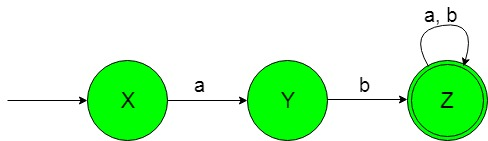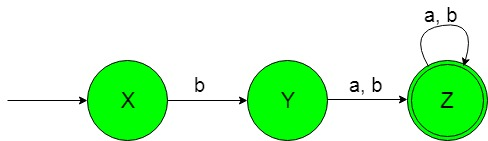# Designing Non-Deterministic Finite Automata (Set 3)

• Last Updated : 18 Jan, 2022

Prerequisite: Finite Automata Introduction
In this article, we will see some designing of Non-Deterministic Finite Automata (NFA).
Problem-1: Construction of a minimal NFA accepting a set of strings over {a, b} in which each string of the language starts with ‘ab’.
Explanation: The desired language will be like:

`L1 = {ab, abba, abaa, ...........}`

Here as we can see that each string of the above language starts with ‘ab’ and end with any alphabet either ‘a’ or ‘b’.
But the below language is not accepted by this NFA because none of the string of below language starts with ‘ab’.

`L2 = {ba, ba, babaaa..............}`

The state transition diagram of the desired language will be like below:In the above NFA, the initial state ‘X’ on getting ‘a’ as the input it transits to a state ‘Y’. The state ‘Y’ on getting ‘b’ as the input it transits to a final state ‘Z’. The final state ‘Z’ on getting either ‘a’ or ‘b’ as the input it remains in the state of itself.

## Python3

 `def` `stateX(n):` `    ``#if length of n become 0 ` `    ``#then print not accepted` `    ``if``(``len``(n)``=``=``0``):` `        ``print``("string ``not` `accepted")` `        `  `    ``else``: ` `        ``#if at zero index ` `        ``#'a' found call` `        ``#stateY function    ` `        ``if` `(n[``0``]``=``=``'a'``):` `            ``stateY(n[``1``:])` `        `  `        ``#if at zero index ` `        ``#'b' then print ` `        ``#not accepted` `        ``elif` `(n[``0``]``=``=``'b'``):` `            ``print``("string ``not` `accepted")   ` `       `  `def` `stateY(n):` `    ``#if length of n become 0 ` `    ``#then print not accepted` `    ``if``(``len``(n)``=``=``0``):` `        ``print``("string ``not` `accepted")` `        `  `    ``else``:  ` `        ``#if at zero index ` `        ``#'a' then print ` `        ``#not accepted   ` `        ``if` `(n[``0``]``=``=``'a'``):` `            ``print``("string ``not` `accepted") ` `            `  `        ``#if at zero index ` `        ``#'b' found call` `        ``#stateZ function    ` `        ``elif` `(n[``0``]``=``=``'b'``):` `            ``stateZ(n[``1``:]) ` `            `  `def` `stateZ(n):` `    ``#if length of n become 0 ` `    ``#then print accepted` `    ``if``(``len``(n)``=``=``0``):` `        ``print``("string accepted")` `        `  `    ``else``:  ` `        ``#if at zero index ` `        ``#'b' found call` `        ``#stateZ function    ` `        ``if` `(n[``0``]``=``=``'a'``):` `            ``stateZ(n[``1``:]) ` `            `  `        ``#if at zero index ` `        ``#'b' found call` `        ``#stateZ function    ` `        ``elif` `(n[``0``]``=``=``'b'``):` `            ``stateZ(n[``1``:])             ` `        `    `            `  `            `  `#take input` `n``=``input``()`   `#call stateA function` `#to check the input` `stateX(n) `

Problem-2: Construction of a minimal NFA accepting a set of strings over {a, b} in which each string of the language is not starting with ‘ab’.
Explanation: The desired language will be like:

`L1 = {ba, bba, bbaa, ...........}`

Here as we can see that each string of the above language is not starting with ‘ab’ but can end with either ‘a’ or ‘b’.
But the below language is not accepted by this NFA because some of the string of below language starts with ‘ab’.

`L2 = {ab, aba, ababaab..............}`

The state transition diagram of the desired language will be like below:In the above NFA, the initial state ‘X’ on getting ‘b’ as the input it transits to a state ‘Y’. The state ‘Y’ on getting either ‘a’ or ‘b’ as the input it transits to a final state ‘Z’. The final state ‘Z’ on getting either ‘a’ or ‘b’ as the input it remains in the state of itself.

## Python3

 `def` `stateX(n):` `    ``#if length of n become 0 ` `    ``#then print not accepted` `    ``if``(``len``(n)``=``=``0``):` `        ``print``("string ``not` `accepted")` `        `  `    ``else``: ` `        ``#if at zero index ` `        ``#'a' found then ` `        ``#print not accepted    ` `        ``if` `(n[``0``]``=``=``'a'``):` `            ``print``("string ``not` `accepted")   ` `        `  `        ``#if at zero index ` `        ``#'b' then call` `        ``#stateY function` `        ``elif` `(n[``0``]``=``=``'b'``):` `            ``stateY(n[``1``:])` `       `  `def` `stateY(n):` `    ``#if length of n become 0 ` `    ``#then print not accepted` `    ``if``(``len``(n)``=``=``0``):` `        ``print``("string ``not` `accepted")` `        `  `    ``else``:  ` `        ``#if at zero index ` `        ``#'a' found call` `        ``#stateZ function   ` `        ``if` `(n[``0``]``=``=``'a'``):` `            ``stateZ(n[``1``:]) ` `            `  `        ``#if at zero index` `        ``#'b' found call` `        ``#stateZ function` `        ``elif` `(n[``0``]``=``=``'b'``):` `            ``stateZ(n[``1``:]) ` `            `  `def` `stateZ(n):` `    ``#if length of n become 0 ` `    ``#then print accepted` `    ``if``(``len``(n)``=``=``0``):` `        ``print``("string accepted")` `        `  `    ``else``:  ` `        ``#if at zero index ` `        ``#'b' found call` `        ``#stateZ function    ` `        ``if` `(n[``0``]``=``=``'a'``):` `            ``stateZ(n[``1``:]) ` `            `  `        ``#if at zero index ` `        ``#'b' found call` `        ``#stateZ function    ` `        ``elif` `(n[``0``]``=``=``'b'``):` `            ``stateZ(n[``1``:])             ` `        `    `            `  `            `  `#take input` `n``=``input``()`   `#call stateA function` `#to check the input` `stateX(n)`

My Personal Notes arrow_drop_up
Recommended Articles
Page :